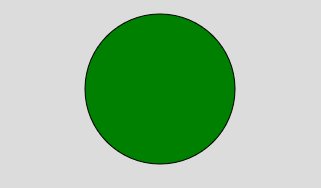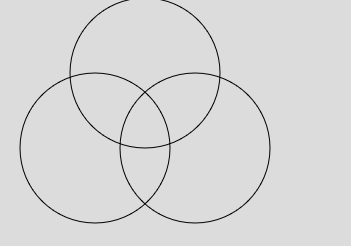# p5.js | circle() Function

The circle() function is used to draw the circle on the screen. A circle is the closed shape. A circle can be created by using the center and radius of the circle.

Syntax:

`circle(x, y, d)`

Parameters:

• x: It is used to set the x-coordinate of the center of the circle.
• y: It is used to set the y-coordinate of the center of the circle.
• d: It is used to set the diameter of the circle.

Example 1:

 `function` `setup() {  ` `     `  `    ``// Create Canvas of given size  ` `    ``createCanvas(400, 300);  ` ` `  `}  ` ` `  `function` `draw() {  ` `     `  `    ``background(220); ` `     `  `    ``// Use color() function ` `    ``let c = color(``'green'``); ` ` `  `    ``// Use fill() function to fill color ` `    ``fill(c); ` `   `  `    ``// Draw a circle  ` `    ``circle(200, 100, 150);  ` `   `  `} `

Output:Example 2:

 `function` `setup() {  ` `     `  `    ``// Create Canvas of given size  ` `    ``createCanvas(400, 300);  ` ` `  `}  ` ` `  `function` `draw() {  ` `     `  `    ``background(220); ` `   `  `    ``// Draw a circle  ` `    ``circle(200, 150, 150);  ` `   `  `    ``noFill(); ` `   `  `    ``// Draw a circle  ` `    ``circle(100, 150, 150);  ` `   `  `    ``// Draw a circle  ` `    ``circle(150, 75, 150); ` `   `  `} `

Output:Reference: https://p5js.org/reference/#/p5/circleMy Personal Notes arrow_drop_upCheck out this Author's contributed articles.

If you like GeeksforGeeks and would like to contribute, you can also write an article using contribute.geeksforgeeks.org or mail your article to contribute@geeksforgeeks.org. See your article appearing on the GeeksforGeeks main page and help other Geeks.

Please Improve this article if you find anything incorrect by clicking on the "Improve Article" button below.

Article Tags :

Be the First to upvote.

Please write to us at contribute@geeksforgeeks.org to report any issue with the above content.